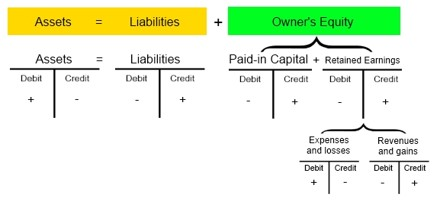# Accounting Equation

Accounting Equation

The source document is the beginning of a transaction and it initiates the accounting procedure, whose starting point is the accounting equation. This is based on dual feature theory (Debit and Credit).

The accounting equation is the formula used to capture the effect of the relationship of financial activities within a business. It emphasizes on the fact that each transaction has a two sided effect i.e., on the assets and claims on assets. Always the total claims (those of outsiders and of the proprietors) will be equal to the total assets of the business concern. The claims are also known as equities, are of two types: i.) Owners equity (Capital); ii.) Outsiders’ equity (Liabilities).

Assets = Equities

Assets = Capital + Liabilities (A = C+L)

Capital = Assets – Liabilities (C = A–L)

Liabilities = Assets – Capital (L = A–C)Example:

If the capital of a business is \$ 3,00,000 and other liabilities are \$ 2,00,000, calculate the total assets of the business.

Solution

Assets = Capital + Liabilities

Capital + Liabilities = Assets

\$  3,00,000 + \$ 2,00,000 = \$5,00,000